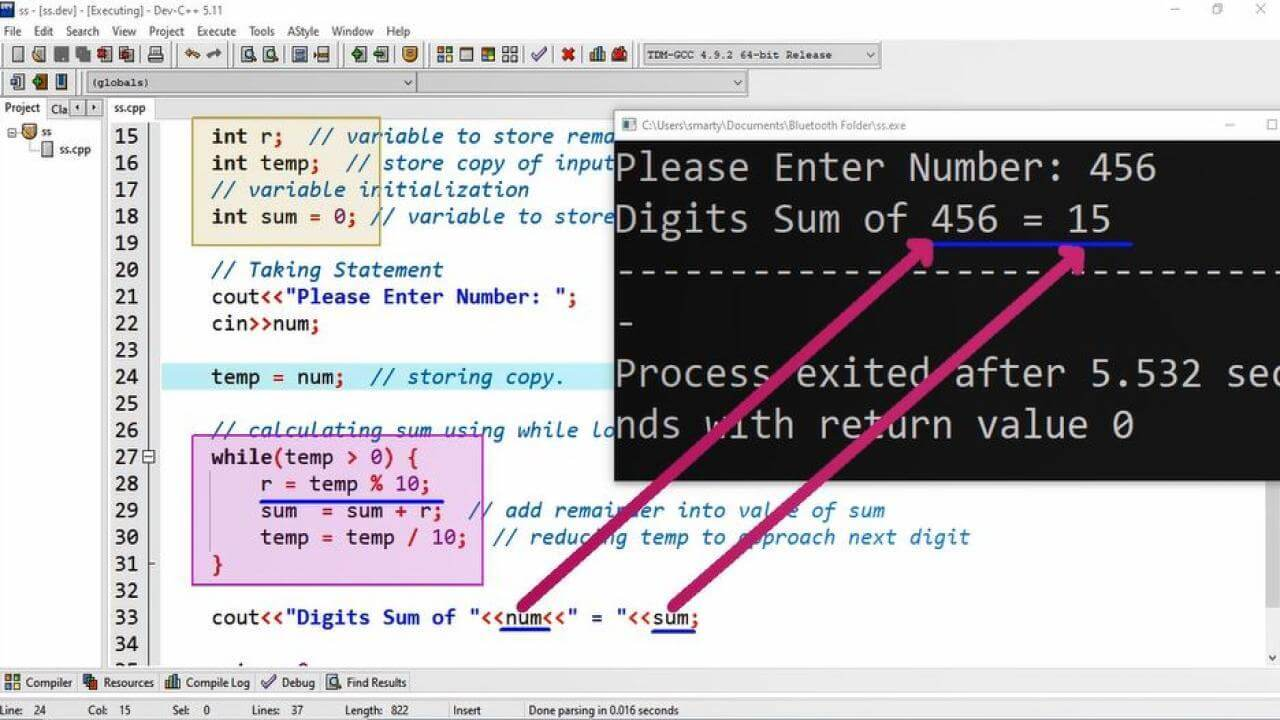# Sum Of Digits Program Example using CPP

## Source Code:

#include <iostream>

/* Program to find sum of
digits of given number
*/

// using standard C++ namespace
using namespace std;

// main function of program
int main() {  // starting delimeter of main function

// variable declarations.
int num;  // variable to take input
int r;  // variable to store remainder temporarily
int temp;  // store copy of input.
// variable initialization
int sum = 0; // variable to store sum

// Taking Statement
cin>>num;

temp = num;  // storing copy.

// calculating sum using while loop
while(temp > 0) {
r = temp % 10;
sum  = sum + r;  // add remainder into value of sum
temp = temp / 10;  // reducing temp to approach next digit
}

cout<<"Digits Sum of "<<num<<" = "<<sum;

return 0;
}

## Output:## Working:

Number is a combination of digits from particular number system. We can say that any number is a set of digits. These digits belongs from specific number system. In this program example we will take input of integer number from user. After taking input will analyze the number using while loop. To analyze any number with respect to its digits the following steps are most important to understand.

1. Check if Number N is greater than 0
2. Take remainder of N
3. Reduce N by dividing it by its base (Radix of numbers system)
4. Repeat from Step 1.

Here we have declare an additional variable named as temp. This temp variable is used to store an  additional copy of input value. The reason is that we will use this temp variable in our computation. And the computation process will reduce this number to 0. After completion of getting digits and their sum, the value of temp variable will be 0. So that we will not lost our orignal input value.

At the end of program we will display orignal number using num variable and its sum using sum variable. In while loop we are adding up each calculated remainder in value of sum variable. The identity of sum /addition is 0 so that we initialze this variable with zero.